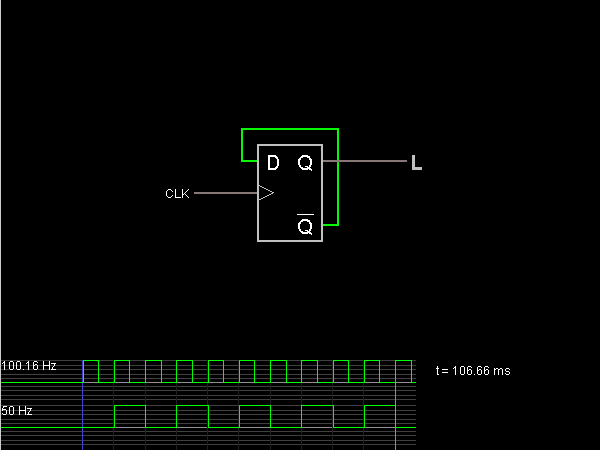# Circuit Simulator - Divide-by-2

## Where can I get Divide-by-2 Circuit Diagram with Explanation?

IndiaBIX provides you lots of fully solved Divide-by-2 circuit diagram with detailed explanation and working principles.

## How to design a Divide-by-2 (electronic circuit)?

You can easily design the Divide-by-2 circuit by practicing the exercises given below. Here you can design and simulate your own electronic circuits with this Online Circuit Designer and Simulator.### Circuit Description:

This circuit shows how a D flip-flop can be used to divide the frequency of a clock signal by 2. -- Credits: Mr. Paul Falstad.